### MEMO 2011 pojedinačno problem 3

Kvaliteta:
Avg: 3,5
Težina:
Avg: 6,6
In a plane the circles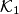$\mathcal K_1$ and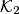$\mathcal K_2$ with centers$I_1$ and$I_2$, respectively, intersect in two points$A$ and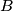$B$. Assume that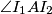$\angle I_1AI_2$ is obtuse. The tangent to$\mathcal K_1$ in$A$ intersects$\mathcal K_2$ again in$C$ and the tangent to$\mathcal K_2$ in$A$ intersects$\mathcal K_1$ again in$D$. Let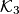$\mathcal K_3$ be the circumcircle of the triangle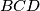$BCD$. Let$E$ be the midpoint of that arc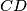$CD$ of$\mathcal K_3$ that contains$B$. The lines$AC$ and$AD$ intersect$\mathcal K_3$ again in$K$ and$L$, respectively. Prove that the line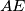$AE$ is perpendicular to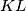$KL$.
Izvor: Srednjoeuropska matematička olimpijada 2011, pojedinačno natjecanje, problem 3